# Roman Numerals 1 to 10

Roman Numerals 1 to 10#### Roman Numerals Chart 1 to 10• This is a list of the Roman numerals 1 to 10.
• I is the Roman numeral for 1.
• V is the Roman numeral for 5.
• X is the roman numeral for 10.
• We can use any of the numerals I, V or X up to three times in a row.
• We can add a numeral by writing it to the right of another.
• Two ‘I’ numerals is 2 and three ‘I’ numerals is 3.
• We can subtract 1 from 5 or 10 by writing I to the left of V or X.
• 4 is written as IV, which is one before five.
• 9 is written as IX, which is one before ten.

I = 1, V = 5, X = 10.

We can add these numerals together up to 3 times in a row to write other numbers.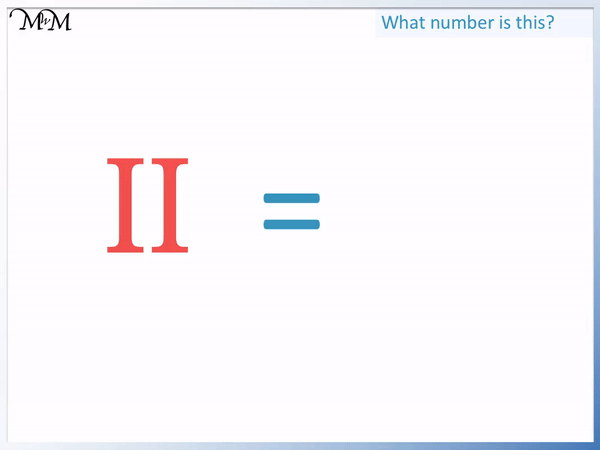• I is the roman numeral for 1.
• We can add multiple I numerals together by writing them next to another.
• We have two I numerals, which is 1 + 1.
• II is worth 2 in Roman numerals.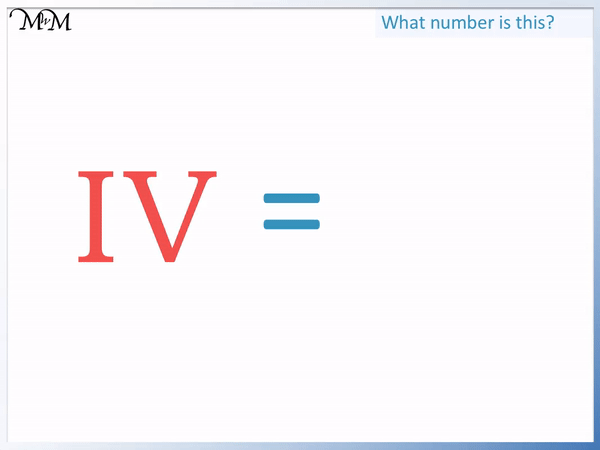• I is worth 1 and V is worth 5.
• We can subtract from V by writing I before it.
• We have an I before a V which means 1 before 5.
• 1 before 5 is 4.
• IV is worth 4.#### Roman Numerals 1 to 10 Interactive Question Generator

Roman Numerals 1 to 10: Interactive Questions

# Roman Numerals 1 to 10 Explained

## What are Roman Numerals

Roman numerals are a different system of writing numbers. Instead of using the digits of 1, 2, 3, 4, 5, 6, 7, 8, 9 or 0, Roman numerals use the capital letters of the alphabet: I, V, X, L, C, D and M.

To write larger numbers, numerals can be added by writing them next each other.

Roman numerals to 10 are written using the three numerals of I, V and X.

I is the first Roman numeral. I is the roman numeral for 1.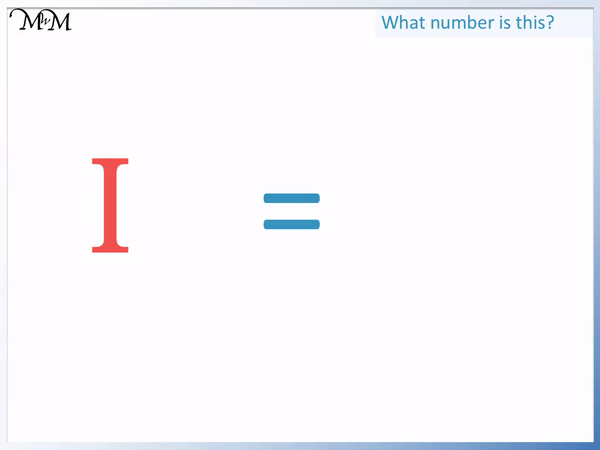We can add two ones together to make 2. We add numerals by writing them next to each other.

II is made from two I numerals. II means 1 + 1.II is worth 2.

2 is written as II in Roman numerals.We can add up to three of each numeral.

We can add three I numerals to make III.

III means 1 + 1 + 1.

3 is written as III in Roman numerals.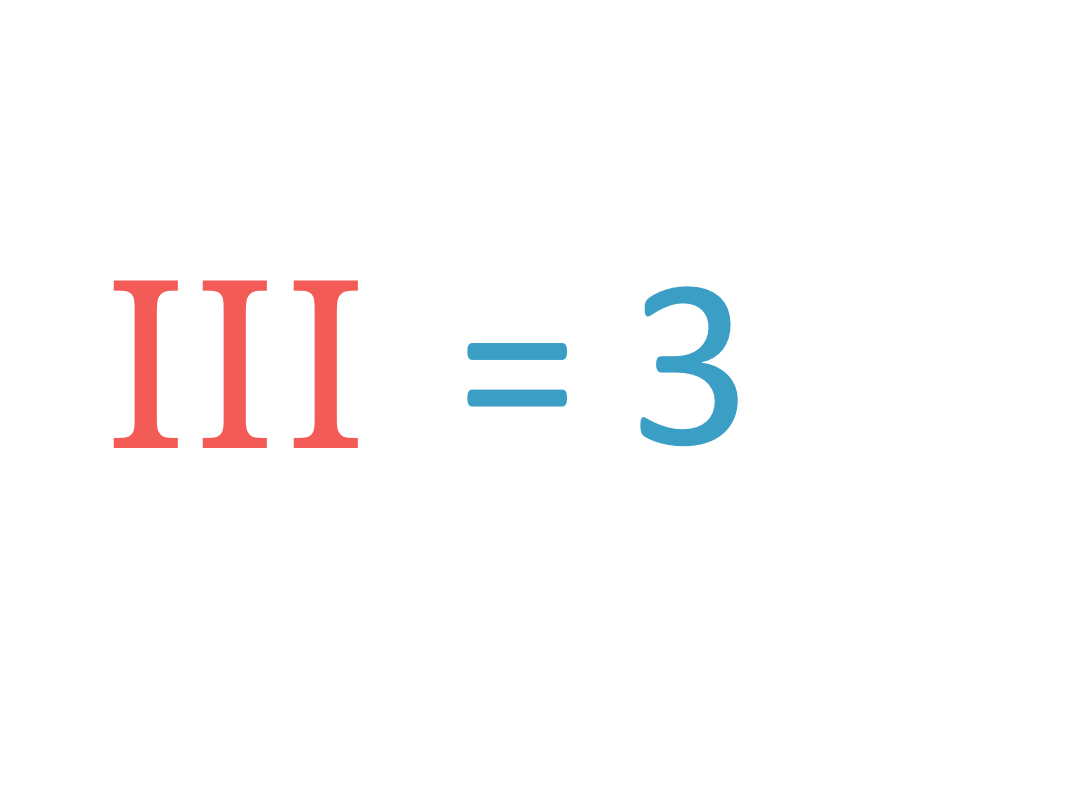We can only use three Roman numerals together at once.

We have already used three I numerals to make III.

To make larger numbers, we need to use the numeral of V.

V is the Roman numeral for 5.So far we have:

I = 1

II = 2

III = 3

V = 5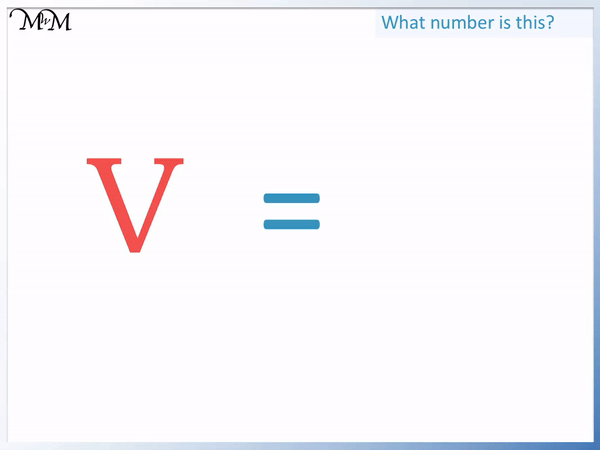4 is written a little differently in Roman numerals.

We are not supposed to write more than 3 of the same numeral together. We can’t write IIII for 4 because this contains more than three I numerals.

Instead we use the fact that 4 is one less than 5.

We can write 4 as one less than five.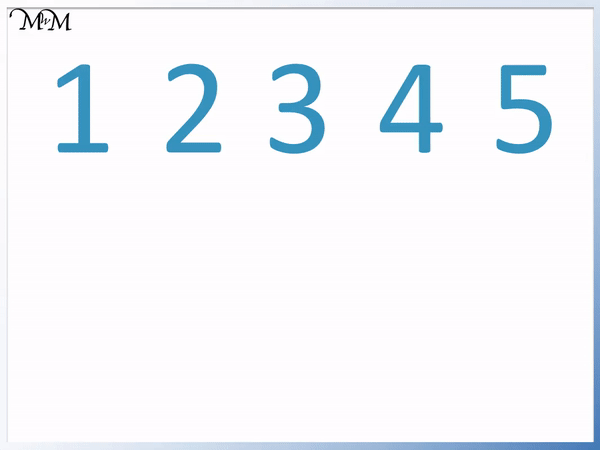We write 4 as one before five. 1 is written as ‘I’ and 5 is written as ‘V’.

To write 1 before 5, we write I before V.4 is written as IV in Roman numerals.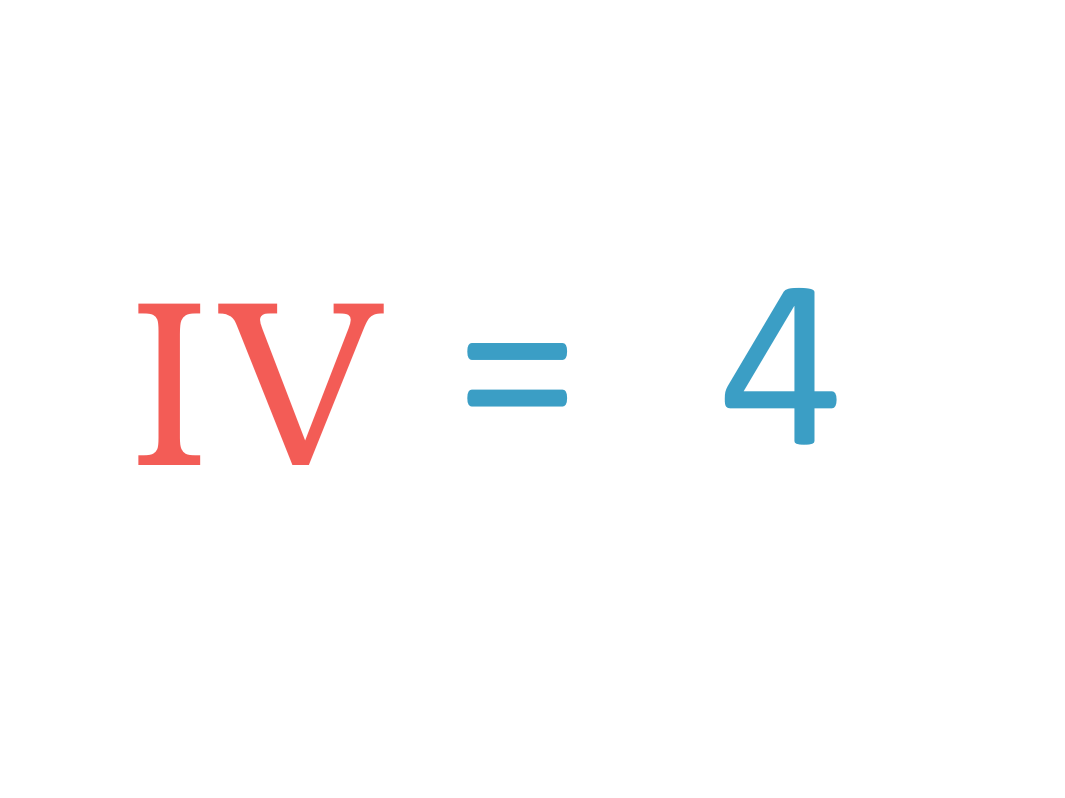Here is a chart showing the Roman numerals to 5.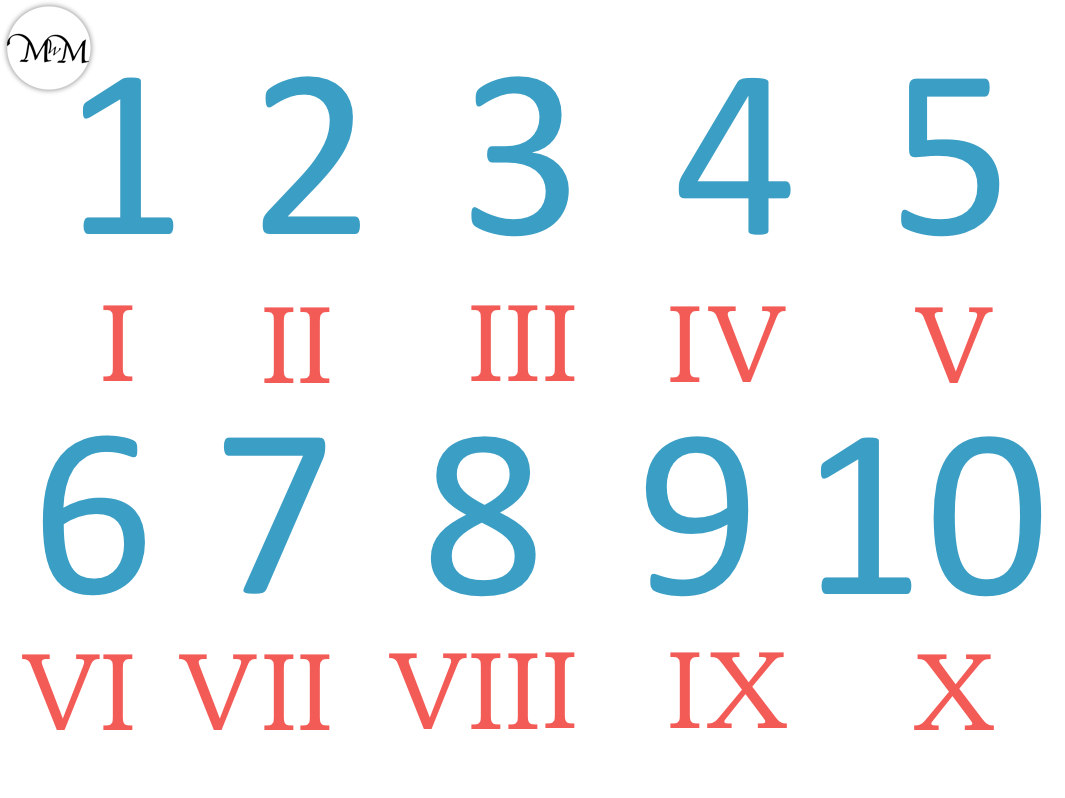We have I for 1, II for 2, III for 3, IV for 4 and V for 5.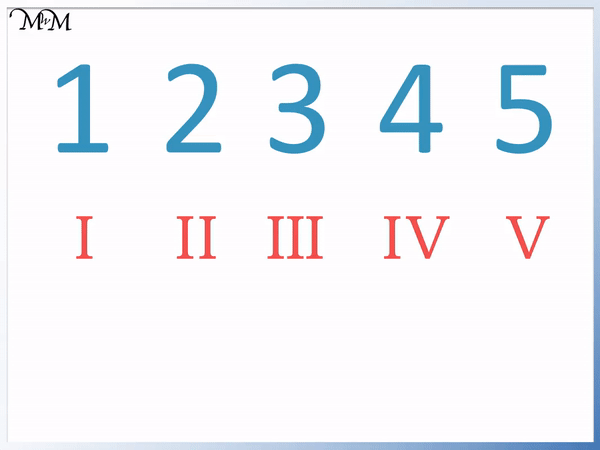To make the numbers larger than 5 using Roman numerals, we count on in ones from five.

5 is written using the numeral of V.

We can count on from five using the numeral of I. We can use up to three I numerals at once.

6 is one more than five. To write one more than five, we write an I after a V.

6 is Roman numerals is VI. VI is V + I, which is 5 + 1.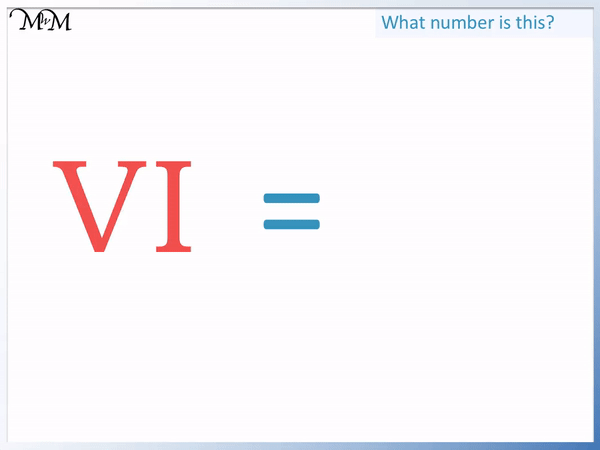7 is two more than five. To write two more than five, we write two I numerals after a V numeral.

7 in Roman numerals is VII. VII is V + I + I, which is 5 + 1 + 1.

8 is three more than five. To write three more than five, we write three I numerals after the V numeral.

8 in Roman numerals is VIII. VIII is V + I + I + I, which is 5 + 1 + 1 + 1.The Roman numeral for 10 is X.In the same way that we wrote 4 as IV, we write 9 as IX.We cannot write VIIII for 9 because it would mean using four I numerals. We are only allowed to use three at most.

We write 9 in Roman numerals as IX, which is an I before an X. 1 before 10 is 9.

Both 4 and 9 are different to the other Roman numerals because they have an I before another numeral.

4 is one before 5 and so is written IV.

9 is one before 10 and so is written IX.

These are the only numbers in the list of Roman numerals to 10 that behave like this.

When teaching Roman numerals, 4 and 9 are most commonly written incorrectly.

Some students can think that IV is 1 + 5, rather than reading it as 1 before 5.

It is important to show the difference between 4 and 6.

4 is IV and 6 is VI. If a smaller numeral is written before a larger value numeral then we subtract the smaller numeral away from the larger numeral.

There is no Roman numeral for zero.Now try our lesson on Roman Numerals to 20 where we learn how to read and write Roman numerals up to 20.